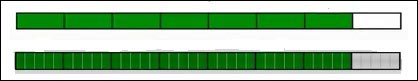# Equivalent Fractions

Equivalent fractions are different fractions that have the same value. The equivalent fractions of a given fraction are those fractions, whose numerators and denominators are in the same ratio as that of the original fraction.

Example: 1/3, 2/6, 3/9, 4/12..are equivalent fractions.

Equivalent fraction of a given fraction is got by multiplying or dividing its numerator and denominator by the same whole number.

For example, if we multiply the numerator and denominator of 2/3 by 4 we get.

2/3 = (2 × 4) / (3 × 4) = 8/12 which is an equivalent fraction of 2/3.

Similarly, if we divide the numerator and denominator of 12/18 by 6 we get.

12/18 = (12 ÷ 6) / (18 ÷ 6) = 2/3. So 12/18 is an equivalent fraction of 2/3 as well.

Fill in the blank to make the fractions equivalent.

7/8 =? /40

### Solution

Step 1:

To get an equivalent fraction, we multiply the numerator and the denominator by the same number

To go from 7/8 to ?/40, we multiply the denominator and numerator with 5 as follows.

7/8 = (7 × 5) / (8 × 5) = 35/40.

Step 2:

So we have filled the blank with 7 × 5 = 35.

So from 7/8 = 35/40, 35 is the answer.

7/8 and 35/40 are equivalent fractions.

Step 3:

We can check the answers with the pictures below

Below 7/8 parts are shaded. Splitting each part into 5 equal parts, we see that 35/40 of the smaller parts are shaded.Fill in the blank to make the two fractions equivalent.

4/5 = ?/20

### Solution

Step 1:

To go from 4/5 to ?/20, we multiply the denominator and numerator with 4 as follows.

4/5 = (4 × 4) / (5 × 4) = 16/20.

Step 2:

So we have filled the blank with 4×4 = 16.

So from 4/5 = 16/20, 16 is the answer

4/5 and 16/20 are equivalent fractions

Fill in the blank to make the two fractions equivalent.

?/28 = 3/4

### Solution

Step 1:

To go from 3/4 to ?/28, we multiply the denominator and numerator with 7 as follows.

3/4 = (3 × 7) / (4 × 7) = 21/28.

Step 2:

So we have filled the blank with 3×7 = 21.

So from 21/28 = 3/4, 21 is the answer.

3/4 and 21/28 are equivalent fractions.